Block and Tackle A block and tackle is made up of multiple pulleys, some of them fixed and some movable. In Figure 3-12, the block and tackle is made up of four pulleys, the top two being fixed and the bottom two being movable.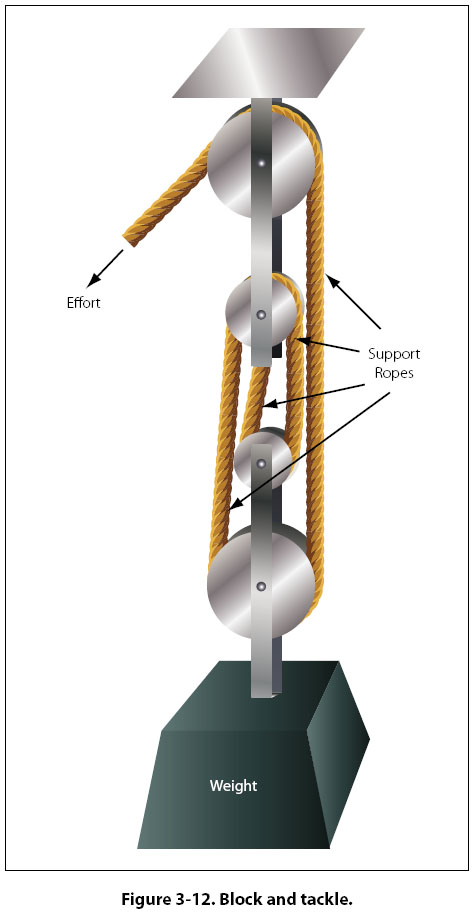Viewing the figure from right to left, notice there are four ropes supporting the weight and a fifth rope where the effort is applied. The number of weight supporting ropes determines the mechanical advantage of a block and tackle, so in this case the mechanical advantage is four. If the weight was 200 lb, it would require a 50 lb effort to lift it. The Gear Two gears with teeth on their outer edges, as shown in Figure 3-13, act like a first class lever when one gear drives the other. The gear with the input force is called the drive gear, and the other is called the driven gear. The effort arm is the diameter of the driven gear, and the resistance arm is the diameter of the drive gear.Notice that the two gears turn in opposite directions (the bottom one clockwise and the top one counterclockwise). The gear on top (yellow) is 9 inches in diameter and has 45 teeth, and the gear on the bottom (blue) is 12 inches in diameter and has 60 teeth.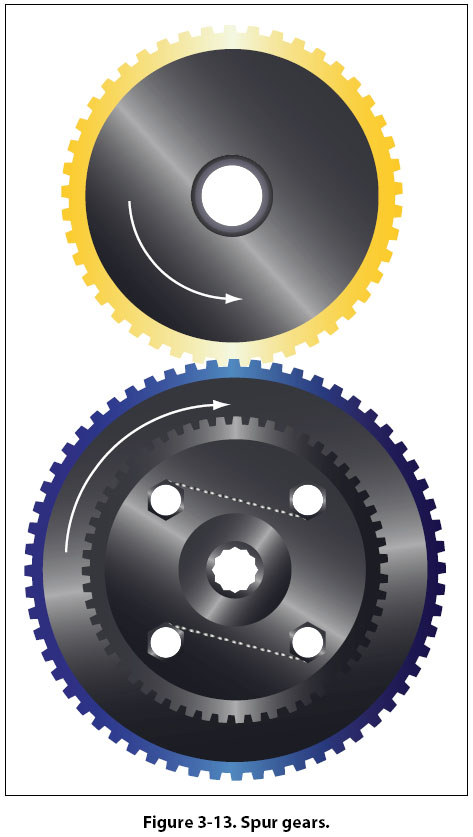Imagine that the blue gear is driving the yellow one (blue is the drive, yellow is the driven). The mechanical advantage in terms of force would be the effort arm divided by the resistance arm, or 9 ÷ 12, which is 0.75. This would actually be called a fractional disadvantage, because there would be less force out than force in. The mechanical advantage in terms of distance (rpm in this case), would be 12 ÷ 9, or 1.33. This analysis tells us that when a large gear drives a small one, the small one turns faster and has less available force. In order to be a force gaining machine, the small gear needs to turn the large one. When the terminology reduction gearbox is used, such as a propeller reduction gearbox, it means that there is more rpm going in than is coming out. The end result is an increase in force, and ultimately torque. Bevel gears are used to change the plane of rotation, so that a shaft turning horizontally can make a vertical shaft rotate. The size of the gears and their number of teeth determine the mechanical advantage, and whether force is being increased or rpm is being increased. If each gear has the same number of teeth, there would be no change in force or rpm. [Figure 3-14]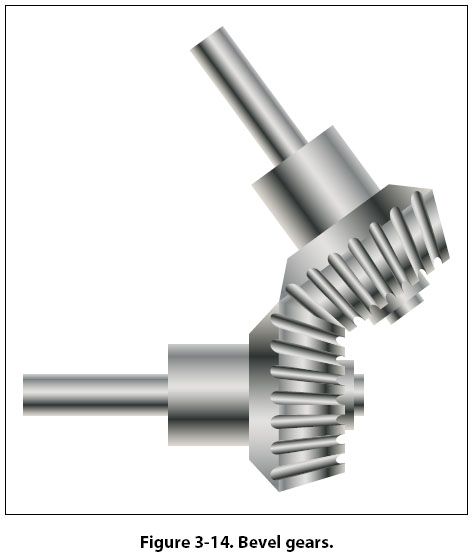The worm gear has an extremely high mechanical advantage. The input force goes into the spiral worm gear, which drives the spur gear. One complete revolution of the worm gear only makes the spur gear turn an amount equal to one tooth. The mechanical advantage is equal to the number of teeth on the spur gear, which in this case is 25. This is a force gaining machine, to the tune of 25 times more output force. [Figure 3-15]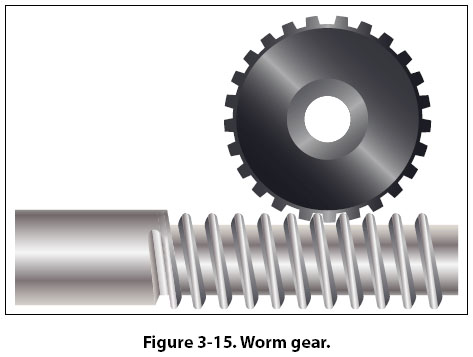The planetary sun gear system is typical of what would be found in a propeller reduction gearbox. The power output shaft of the engine would drive the sun gear in the middle, which rotates the planetary gears and ultimately the ring gear. In this example, the sun gear has 28 teeth, each planet gear has 22 teeth, and the ring gear has 82 teeth. To figure how much gear reduction is taking place, the number of teeth on the ring gear is divided by the number of teeth on the sun gear. In this case, the gear reduction is 2.93, meaning the engine has an rpm 2.93 times greater than the propeller. [Figure 3-16]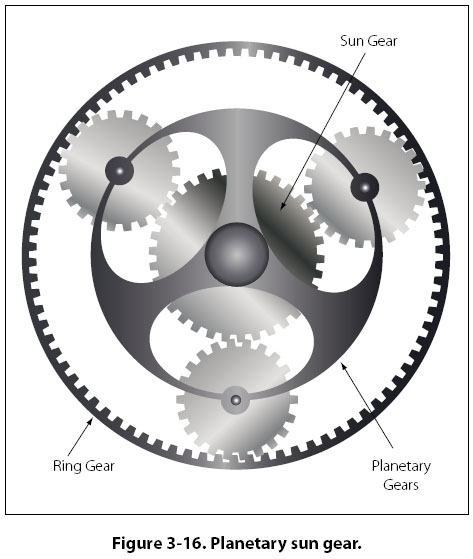©AvStop Online Magazine                                                                                                                                                      Contact Us              Return To Books